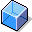Multilevel BOMDefinition

BOM containing materials that have their own BOMs (assemblies)

Use

The system assigns a low-level code (the Logistics view) to each level of the BOM. The following graphic shows a multilevel BOM and its low-level codes:The system explodes the BOM from top to bottom (low-level codes), and assigns costing levels (from the costing view). The following graphic shows a multilevel BOM and its costing levels:The costing levels determine the sequence in which the cost of goods manufactured is calculated for each material.

• The system first calculates the costs for the materials with the lowest costing level (0 in the graphic).
• Then it calculates the costs for the materials (semifinished products) in the next-highest level (1) including the costs that it calculated for the materials in the subordinate costing level.

This ensures that the costs for all assemblies and all raw materials or purchased parts are included in the costs calculated for the end product. This process is also called cost rollup. A cost component split is created for each assembly costed, grouping the costs into, for example, material costs, production costs, and overhead. For raw materials or purchased parts, you can create an initial cost split to break down the procurement costs.

When you save the cost estimate for the end product, a cost component split for each level of the cost estimate is saved as well. This enables you to analyze the value added at each production level.